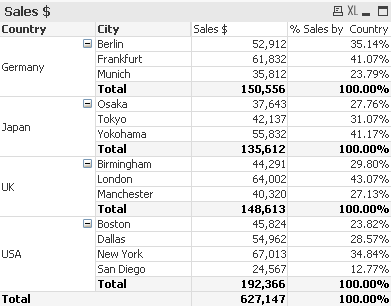# QlikView Scripting

Discussion Board for collaboration on QlikView Scripting.

Announcements

#### Breathe easy -- you now have more time to plan your next steps with Qlik! QlikView 11.2 Extended Support is now valid through December 31, 2020. Click here for more information.Not applicable

## calculate the percentaje of subtotal

I every one;

I need to get the calculate the percentaje of subtotal like % Sales by Country ColumnMy problem is that I have the condition IF,  where I used the function TOTAL I need your help to put the correct scripting

this is my formula:

% Percentaje =

(if(year_ent=year(today()),conta_ent,0))/
Sum(TOTAL <week_ent,General_Performance > if(year_ent=year(today()),conta_ent,0) )   <--the problem is here in the second line is incorrect the syntaxes can you tell me please?MVP

## Re: calculate the percentaje of subtotal

are you sure the problem is in the second line and not in the first one?

Sum(if(year_ent=year(Today()),  conta_ent, 0) )

/

Sum(TOTAL <week_ent,General_Performance> if(year_ent=year(Today()),  conta_ent, 0) )

Community Browser